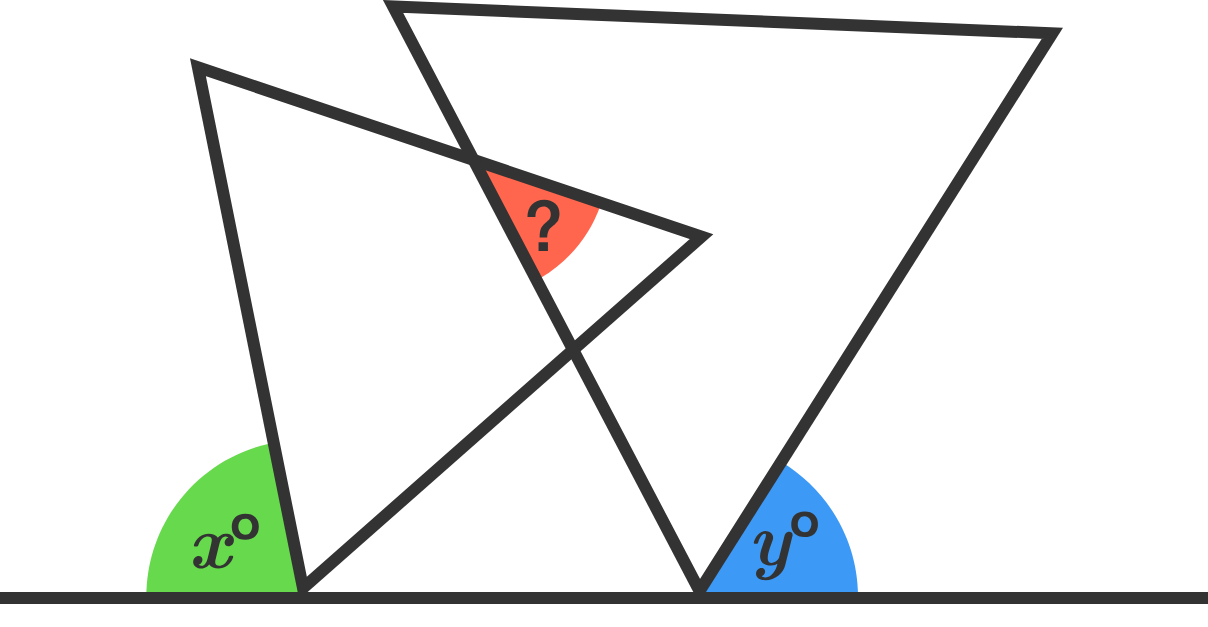# 98 of 100: Triangle Tangle

Geometry Level 1Two equilateral triangles overlap as shown. What is the measure in degrees of the red angle?As usual for the geometry problems in this challenge, there are many ways to approach this problem.

×